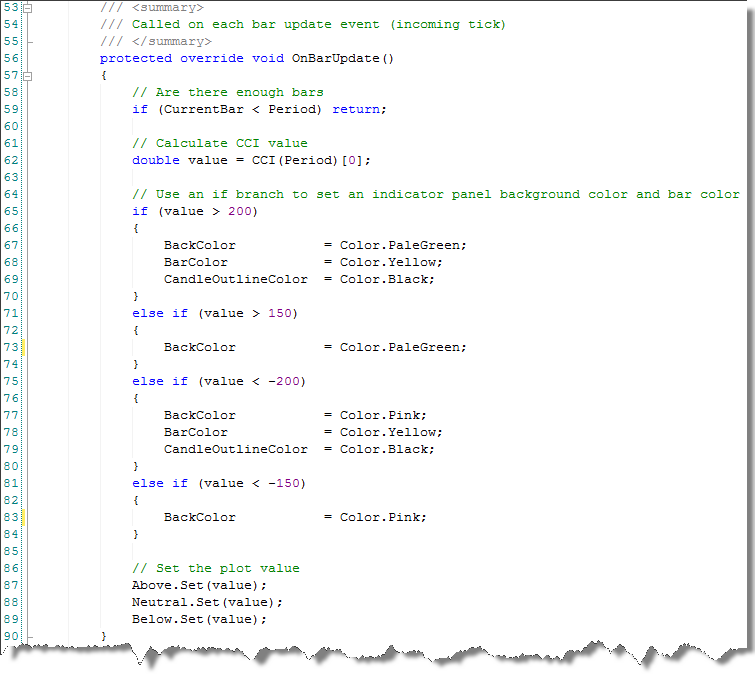﻿ Entering Calculation Logic
 NinjaScript > Educational Resources > Tutorials > Indicators > Advanced - Custom Drawing > Entering Calculation Logic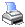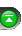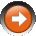The OnBarUpdate() method is called for each incoming tick or on the close of a bar (user defined) when performing real-time calculations and is called on each bar of a data series when re-calculating the indicator. For example, an indicator would be re-calculated when adding it to an existing chart that has existing price data displayed. Therefore, this is the main method called for indicator calculation and we will use this method to enter the script that will calculate the CCI value and set the conditions used to set custom drawing.

Initializing the Indicator
The Initialize() method is called once before any bar data is loaded and is used to configure the indicator. The code below is automatically generated by the wizard and added to the Initialize() method. It configures the indicator for three plots and two lines and sets the parameters.

Enter the following code in the Initialize() method and below the wizard generated code:

 // Set the pen used to draw the plot as a dashed line Plots.Pen.DashStyle = DashStyle.Dash;

The third plot named "Below" is of color OrangeRed and has a PlotStyle of line. The code above changes the DashStyle property of this line from a solid line to a dashed line. This is accomplished by accessing the plot via Plots syntax. New plots are always added to the collection Plots in sequence. Based on the wizard generated code above, we can see that the plot named "Below" was the third added plot. Since collections are always zero based we pass in the value of 2 (plot 1 = 0, plot 2 = 1, plot 3 = 2 etc...) to then access the Pen used to draw the plot and change the DashStyle property. This is an advanced topic. Changing this property can be done by the user of the indicator directly within the Indicator Dialog window. You can read more about the Pen class here.

Enter the following code in the Initialize() method and below the code entered above:

 // Set line drawing thresholds which give the visual effect of // multi colored lines based on indicator values Plots.Min = 70; Plots.Max = 70; Plots.Min = -70; Plots.Max = -70;

The code above sets the plot threshold values. The concept of setting threshold values is to set when and when not to paint a plot on the chart. This topic was covered in greater detail in the "Custom Plot Colors via Thresholds" Tutorial.

Custom Drawing
Replace the wizard generated code with the following code into the OnBarUpdate() method in the NinjaScript Editor:

 // Do not calculate if we don't have enough bars if (CurrentBar < Period) return;   // Calculate CCI value double value = CCI(Period);   // Use an if branch to set an indicator panel back ground color and bar color if (value > 200) {     BackColor          = Color.PaleGreen;     BarColor           = Color.Yellow;     CandleOutlineColor = Color.Black; } else if (value > 150) {     BackColor          = Color.PaleGreen; } else if (value < -200) {     BackColor          = Color.Pink;     BarColor           = Color.Yellow;     CandleOutlineColor = Color.Black; } else if (value < -150) {     BackColor          = Color.Pink; }

The method first checks to ensure that there are enough bars to perform the calculation and then assigns the CCI value to the variable value. A multi case if statement is used to determine when to draw custom graphics.

if (value > 200)
{
BackColor = Color.PaleGreen;
BarColor = Color.Yellow;

CandleOutlineColor = Color.Black;
}

The first branch checks if the value is greater than 200 and if true, sets the BackColor property to PaleGreen, sets the BarColor property to yellow, and also sets the CandleOutlineColor property to black.

BackColor sets the background color of the chart for the current bar.
BarColor sets the color of the current price bar.

CandleOutlineColor sets the color of the outline of the current price bar.

A list of available colors can be found here.

Each successive else if branch checks the value of value and sets custom drawing properties.

Setting the plot values
Enter the following code in the OnBarUpdate() method and below the code entered above:

 // Set the plot value Above.Set(value); Neutral.Set(value); Below.Set(value);

To complete the indicator you must set the indicator value to each plot.

The Initialize() method in your editor should look identical to the image below: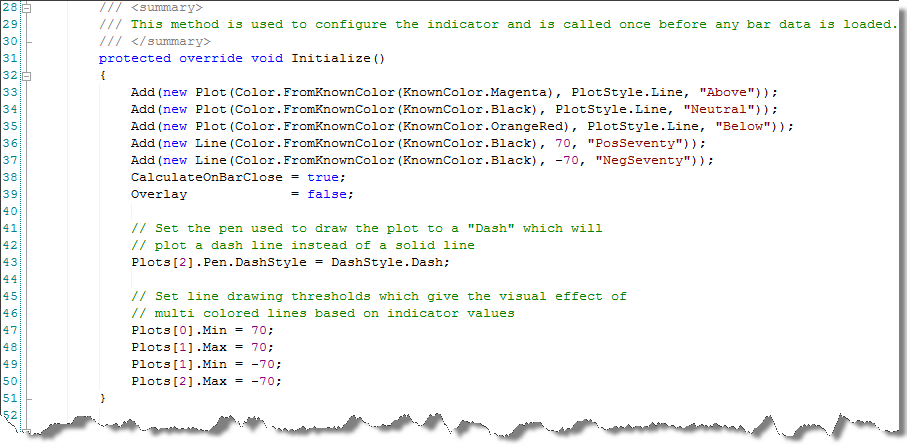The OnBarUpdate() method in your editor should look identical to the image below: